• //--------------提取拟合的直线------------------ /*直线提取方法1 pcl::PointCloud<pcl::PointXYZ>::Ptr c_plane(new pcl::PointCloud<pcl::PointXYZ>); for (int i = 0; i < inliers->...shell
• ggplot2 为图形添加直线
本文更新地址:http://blog.csdn.net/tanzuozhev/article/details/51112057本文在 http://www.cookbook-r.com/Graphs/Scatterplots_(ggplot2)/ 的基础上加入了自己的理解对于连续型数据轴和离散型数据轴# Some sample data
cond result
control     10
treatment   11.5
')

library(ggplot2)# 基础图形
bp <- ggplot(dat, aes(x=cond, y=result)) +
geom_bar(position=position_dodge(), stat="identity")
bp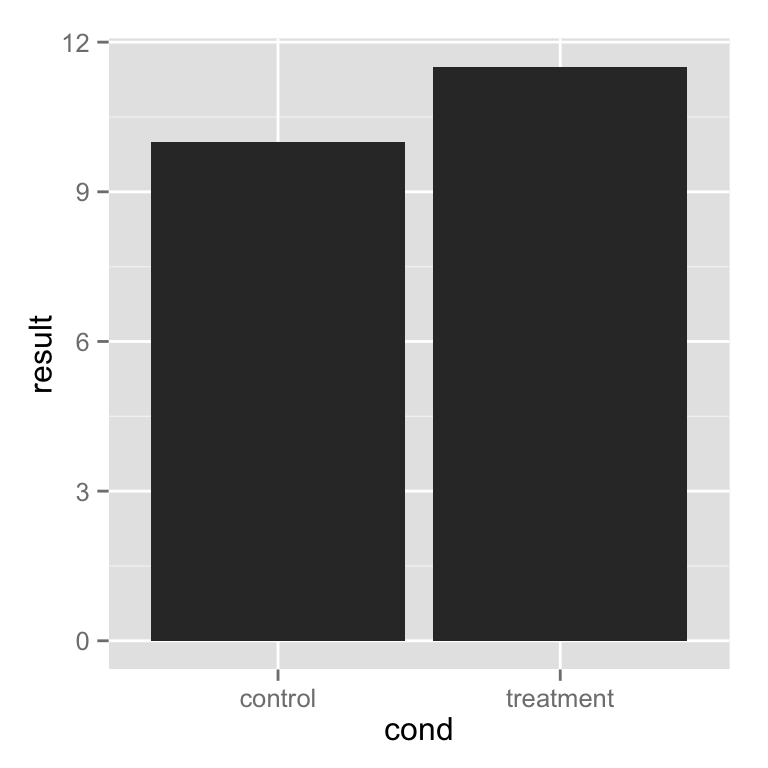# 添加一条水平线
bp + geom_hline(aes(yintercept=12))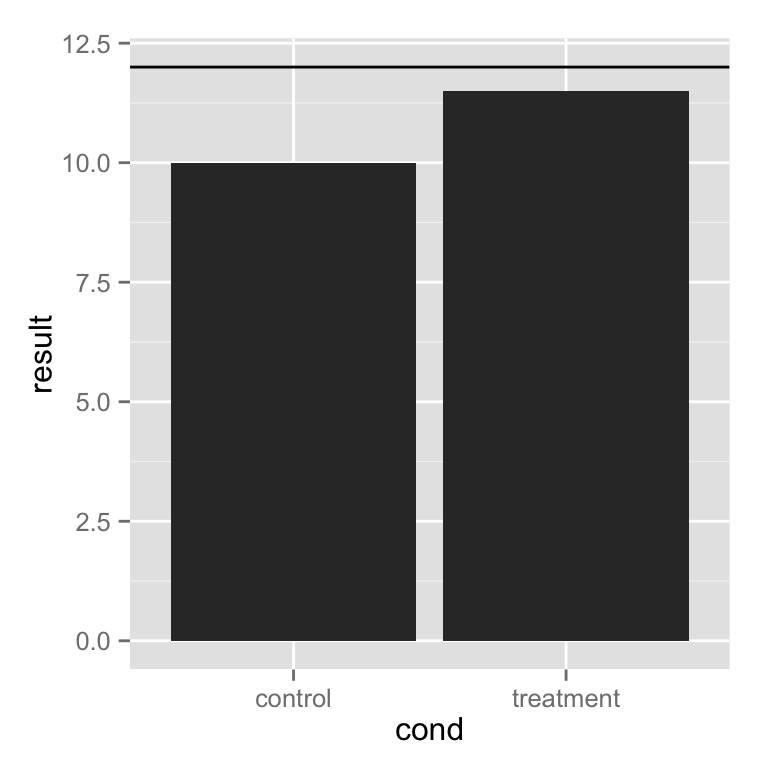# linetype 设置线型为 虚线
bp + geom_hline(aes(yintercept=12), colour="#990000", linetype="dashed")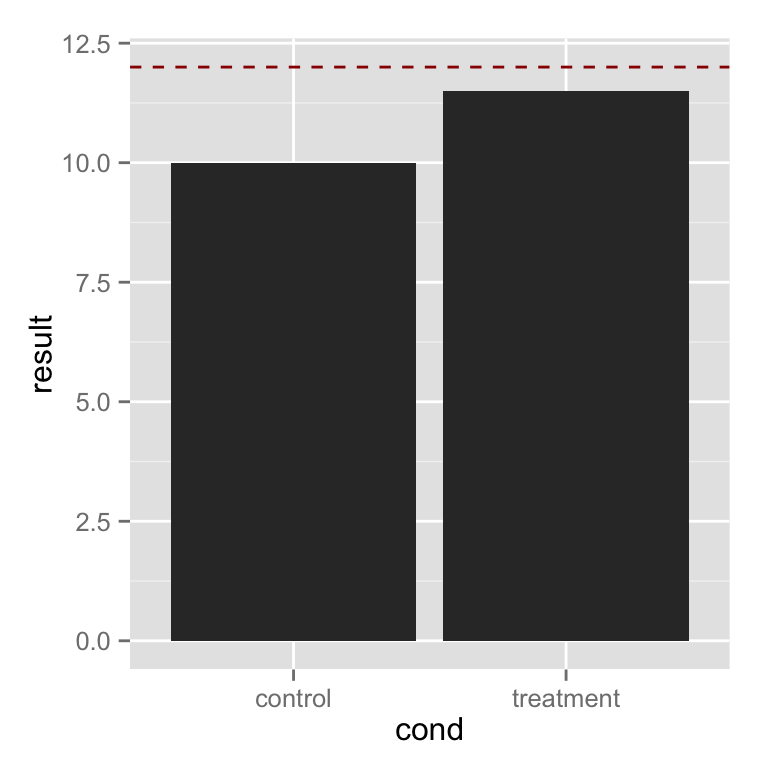根据分类情况,添加直线# Draw separate hlines for each bar. First add another column to dat
dat$hline <- c(9,12) dat## cond result hline ## 1 control 10.0 9 ## 2 treatment 11.5 12# Need to re-specify bp, because the data has changed bp <- ggplot(dat, aes(x=cond, y=result)) + geom_bar(position=position_dodge(), stat="identity") # 对每一个条形添加分割线 bp + geom_errorbar(aes(y=hline, ymax=hline, ymin=hline), colour="#AA0000")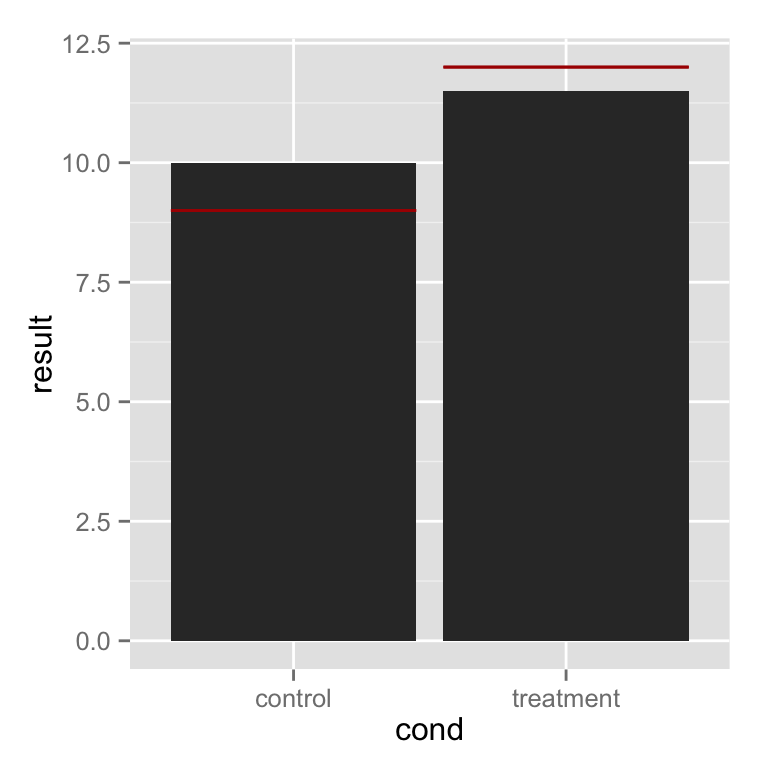bp + geom_errorbar(width=0.5, # 设置线宽度 aes(y=hline, ymax=hline, ymin=hline), colour="#AA0000")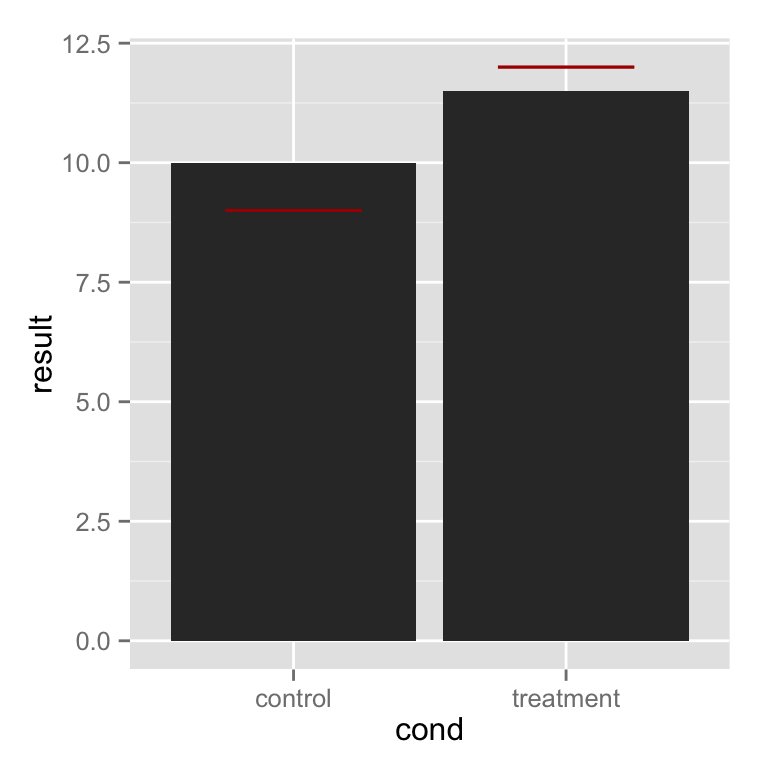# 重新定义数据 dat_hlines <- data.frame(cond=c("control","treatment"), hline=c(9,12)) dat_hlines## cond hline ## 1 control 9 ## 2 treatment 12# 条形图的数据来自于 dat, 但是 线的数据来自于 dat_hlines bp + geom_errorbar(data=dat_hlines, aes(y=hline, ymax=hline, ymin=hline), colour="#AA0000")直线穿过各组数据这里实际上是有4条线, 但是前两条线与后两条线的数值相同,看上去是两条线.dat <- read.table(header=TRUE, text=' cond group result hline control A 10 9 treatment A 11.5 12 control B 12 9 treatment B 14 12 ') dat## cond group result hline ## 1 control A 10.0 9 ## 2 treatment A 11.5 12 ## 3 control B 12.0 9 ## 4 treatment B 14.0 12# Define basic bar plot bp <- ggplot(dat, aes(x=cond, y=result, fill=group)) + geom_bar(position=position_dodge(), stat="identity") bp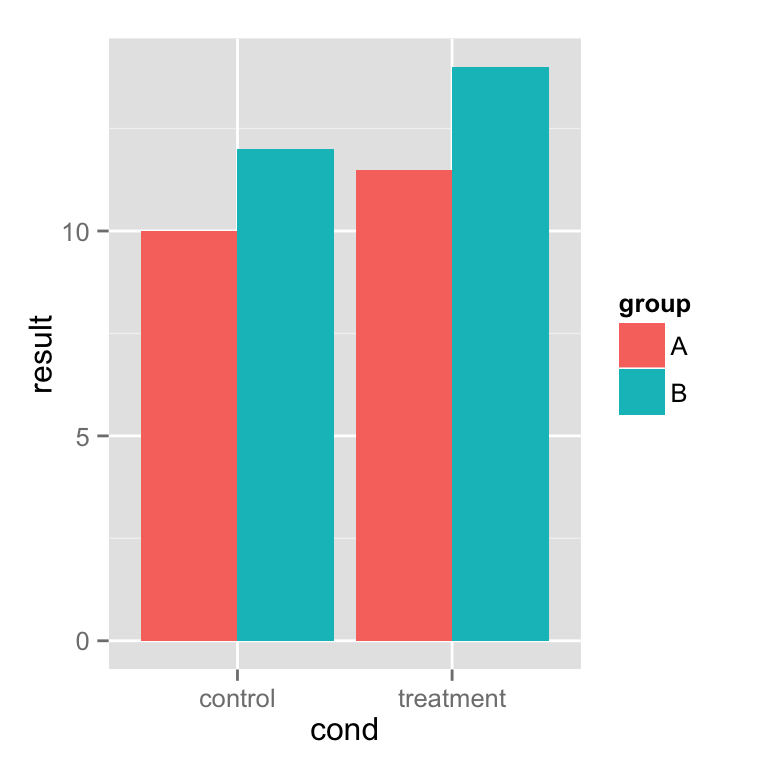# The error bars get plotted over one another -- there are four but it looks # like two bp + geom_errorbar(aes(y=hline, ymax=hline, ymin=hline), linetype="dashed")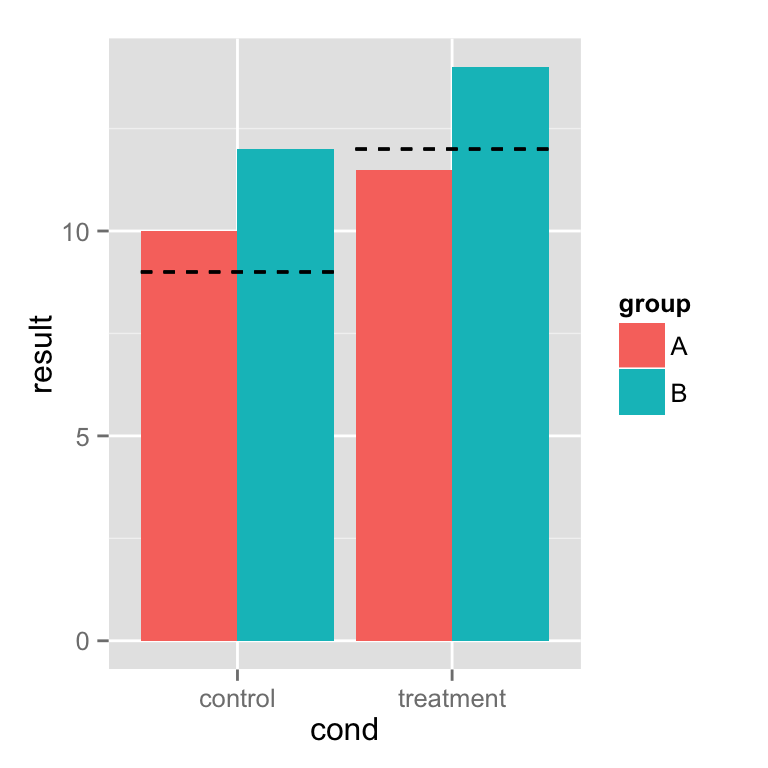每组数据分别划线dat <- read.table(header=TRUE, text=' cond group result hline control A 10 11 treatment A 11.5 12 control B 12 12.5 treatment B 14 15 ') # Define basic bar plot bp <- ggplot(dat, aes(x=cond, y=result, fill=group)) + geom_bar(position=position_dodge(), stat="identity") bpbp + geom_errorbar(aes(y=hline, ymax=hline, ymin=hline), linetype="dashed", position=position_dodge())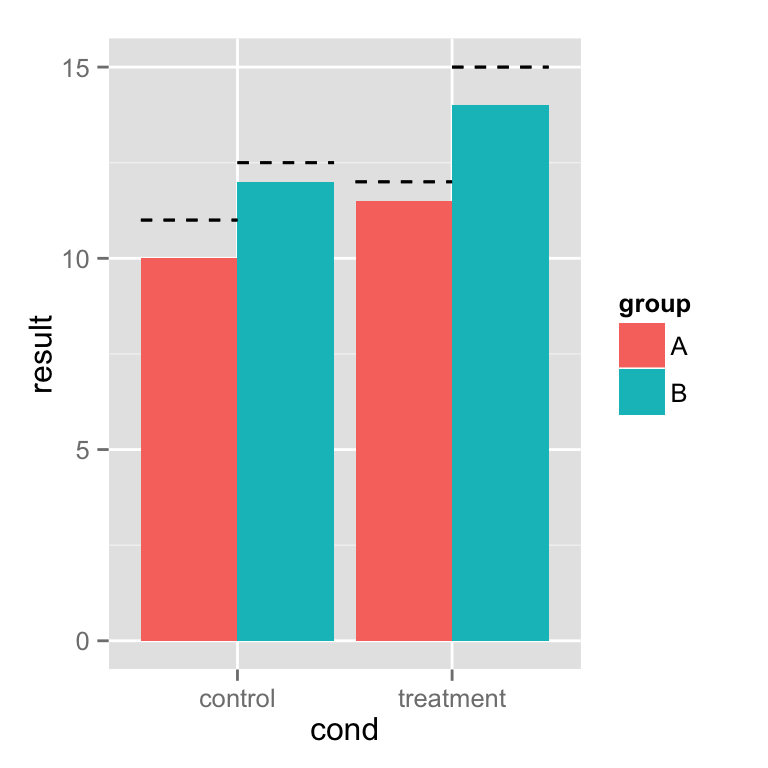为连续型数据轴添加直线dat <- read.table(header=TRUE, text=' cond xval yval control 11.5 10.8 control 9.3 12.9 control 8.0 9.9 control 11.5 10.1 control 8.6 8.3 control 9.9 9.5 control 8.8 8.7 control 11.7 10.1 control 9.7 9.3 control 9.8 12.0 treatment 10.4 10.6 treatment 12.1 8.6 treatment 11.2 11.0 treatment 10.0 8.8 treatment 12.9 9.5 treatment 9.1 10.0 treatment 13.4 9.6 treatment 11.6 9.8 treatment 11.5 9.8 treatment 12.0 10.6 ') library(ggplot2)Basic lines# 基本散点图 sp <- ggplot(dat, aes(x=xval, y=yval, colour=cond)) + geom_point() # 水平线 sp + geom_hline(aes(yintercept=10))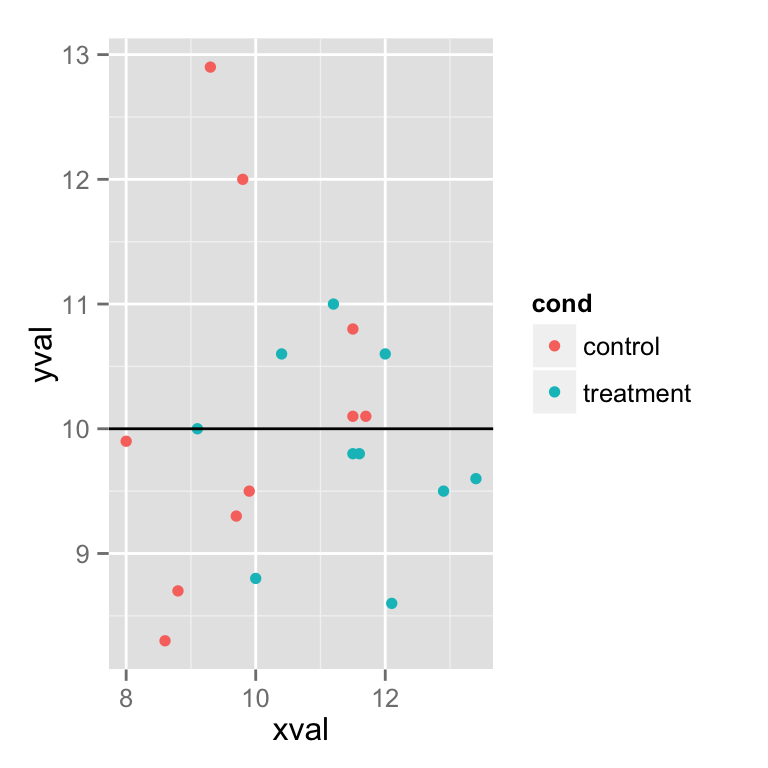# 垂直虚线 sp + geom_hline(aes(yintercept=10)) + geom_vline(aes(xintercept=11.5), colour="#BB0000", linetype="dashed")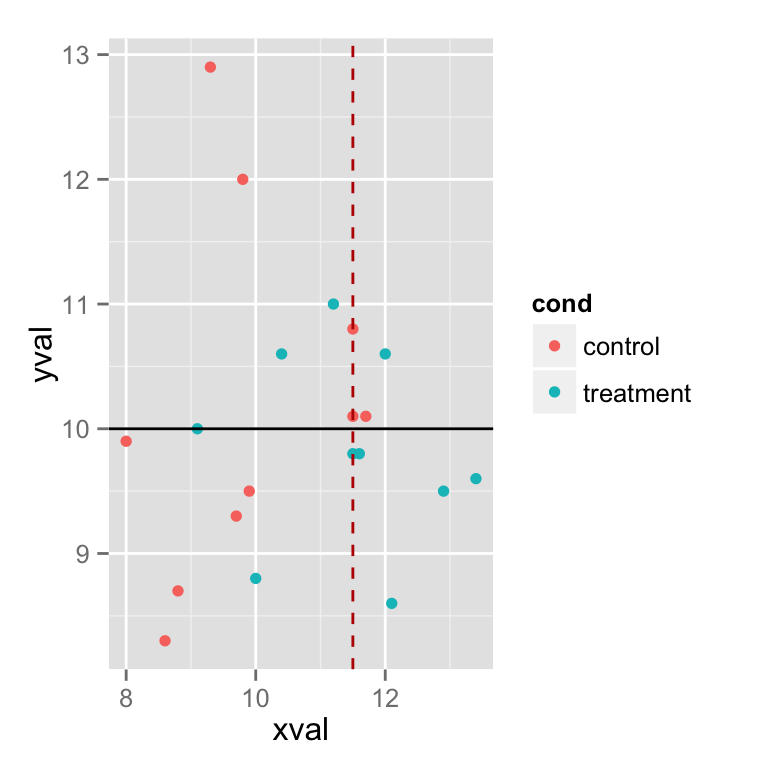为均值自动划线# Add colored lines for the mean xval of each group sp + geom_hline(aes(yintercept=10)) + geom_line(stat="vline", xintercept="mean")# 根据 cond 分面 spf <- sp + facet_grid(. ~ cond) spf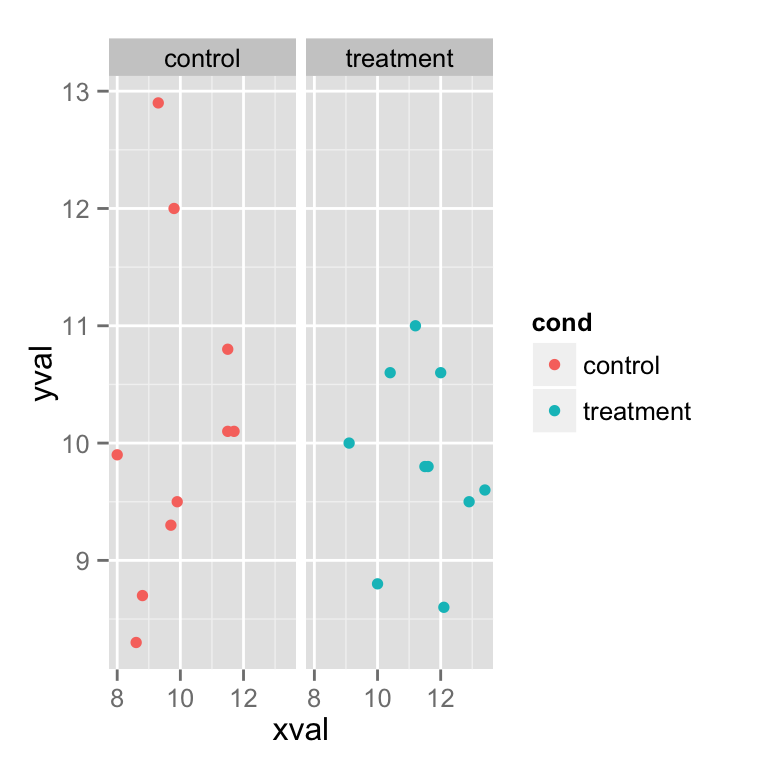# 在所有的面上划线 spf + geom_hline(aes(yintercept=10))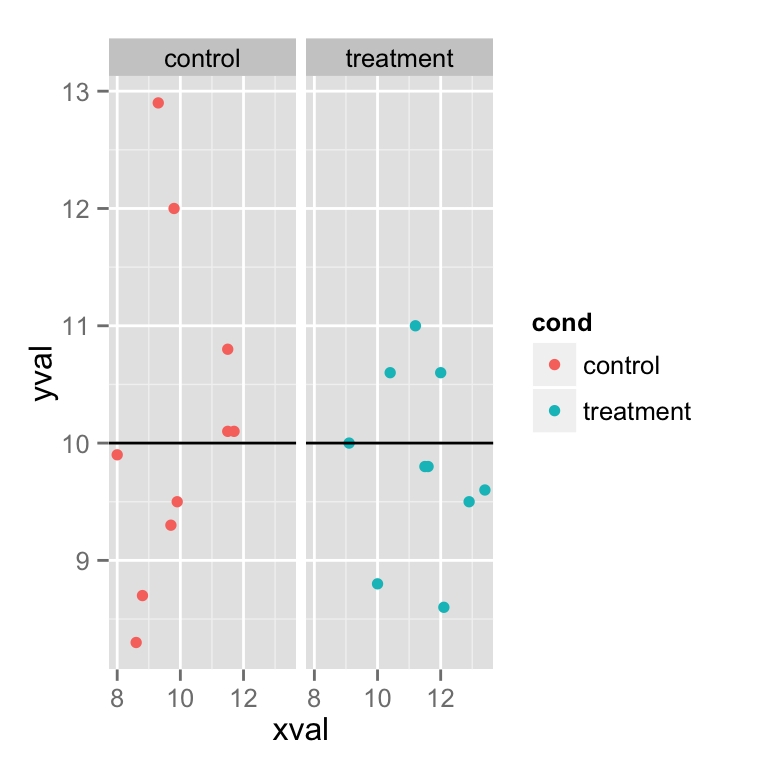如果想为不同的分面, 添加不同的直线, 这里有两种设置. 一种是重新生成一个满足数据需要的 data.frame 另一种是使用 geom_line() 的stat和xintercept# 第一种方法 dat_vlines <- data.frame(cond=levels(factor(dat$cond)), xval=c(10,11.5))
dat_vlines##        cond xval
## 1   control 10.0
## 2 treatment 11.5spf + geom_hline(aes(yintercept=10)) +
geom_vline(aes(xintercept=xval), data=dat_vlines,
colour="#990000", linetype="dashed")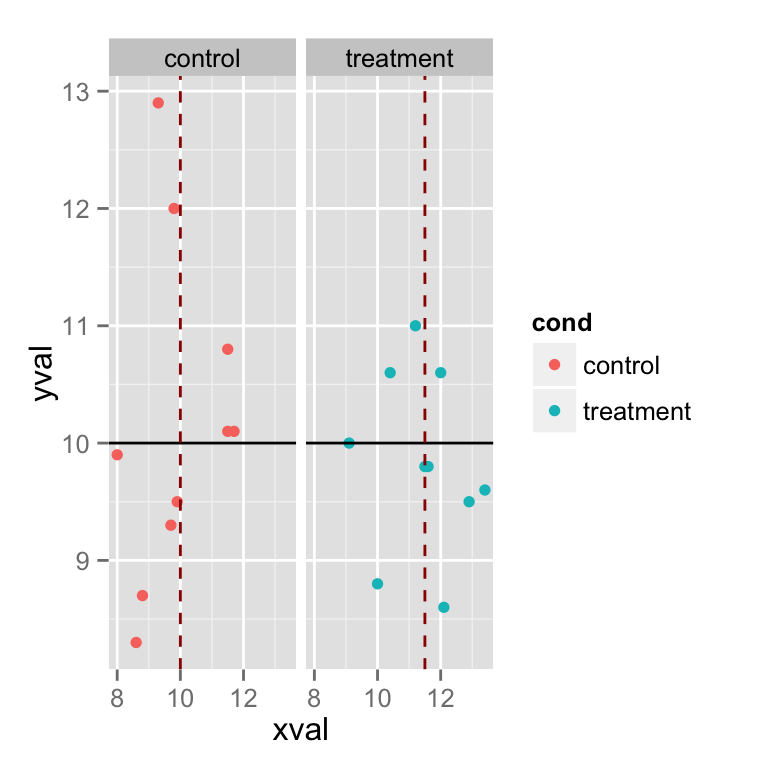# 第二种方法
spf + geom_hline(aes(yintercept=10)) +
geom_line(stat="vline", xintercept="mean")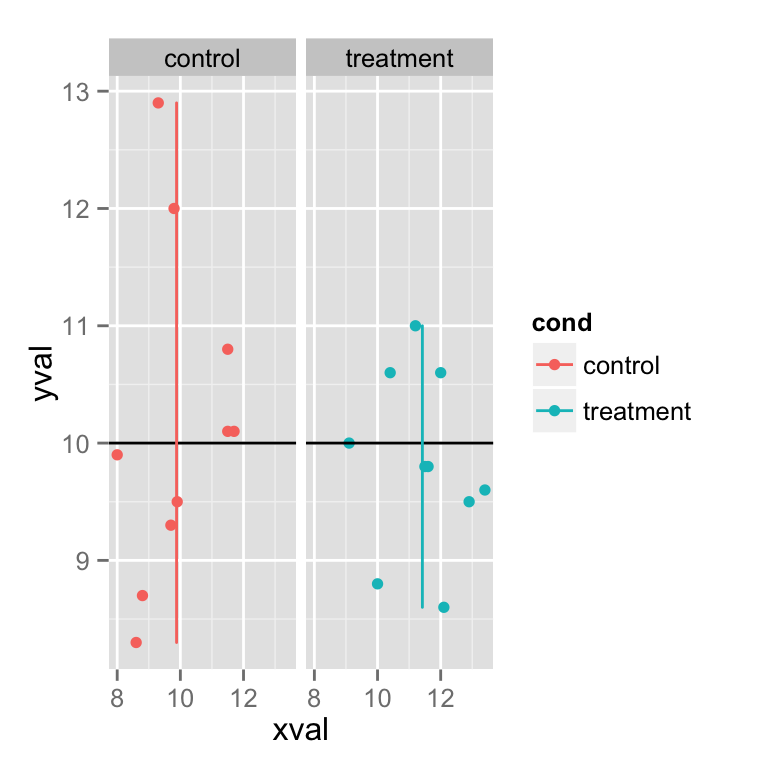展开全文• 直线直线直线与圆、直线与矩形的交点 /*本程序采用java语言，实现直线直线直线与圆、直线与矩形的交点 以（0,0）为坐标原点，建立直角坐标 直线: a*x +b*x +c = 0 参数：a, b, c 圆 参数 ：圆心坐标...
求直线与直线、直线与圆、直线与矩形的交点

直线与直线的交点：a1x + b1x + c1x = 0 与 a2x + b2x + c2x = 0的交点坐标可直接根据公式x = (b1c2 - b2c1)/(a1b2 - a2b1)，y = (a2c1 - a1c2)/(a1b2 - a2b1)得出，其中a1b2 - a2b1 == 0时，代表两条直线重合或平行
直线与圆的交点：通过将圆心到直线的距离d与半径r大小对比分三种情况讨论：1）d>r，没有交点 2）d=r，一个交点 3）d<r，两个交点，具体设计可参考下图（此图是在网上搜方法看到的，原地址找不到了，如有侵犯还请原谅，感谢原主的帮助）：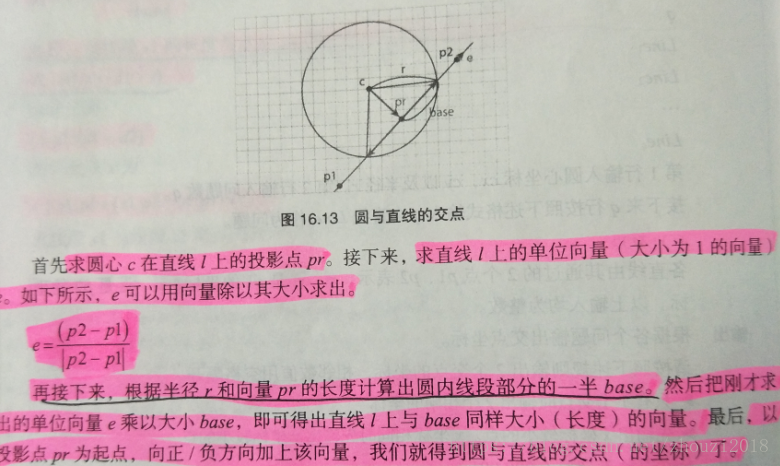直线与矩形的交点：问题可以简化为求直线与构成矩形的四条线段的交点，方法为：假设求直线PQ与线段AB的交点，首先求A到直线PQ的带符号距离d和B到直线PQ的带符号距离d2，如果d1与d2异号或其中至少有一个为0，则交点存在，假设交点为M。D1为A到直线PQ的距离，D2为B到直线PQ的距离，求线段AM占段线AB的比例k=D1/(D1+D2);如果D1+D2==0，即D1和D2均为0，也就是说段线AB与直线PQ重合。有了线段AM点AB的比例k，就可以求出M了： M = A + (B - A) * k;如果d1与d2同号的话就表示直线与线段没有交点。

在以上方法中还有一些需要关注的细节点
1）直线在讨论的过程中需要将直线平行于x,y轴的情况单独讨论
2）求直线与矩形交点时，当交点正好是矩形的顶点时，简单求直线与四条线段的交点会造成顶点在其邻线段被重复计算，因此在计算每条线段时舍去线段的一个端点
代码如下：
/*本程序采用java语言，实现直线与直线、直线与圆、直线与矩形的交点
以（0,0）为坐标原点，建立直角坐标
直线: a*x +b*x +c = 0  参数：a, b, c
圆 参数 ：圆心坐标（x0, y0）  半径 r
矩形参数：三个顶点坐标A:（x1, y1）B:（x2, y2）C:（x3, y3）  限制∠ABC构成直角*/

package robotcup;

public class GetIntersection {

public static void main(String[] args) {
// TODO Auto-generated method stub
pointTwoLine(1, 1, -1, 1, -1, 0);//求两直线交点   直线一：x+y-1=0  直线二：x-y=0
pointLineCircle(1, 1, -1, 1, 1, 1);//求直线与圆的交点  直线：x+y-1=0     圆心:(1,1) r=1
pointLineRectangular(1, 1, -0.5, 0, 1, 0, 0, 1, 0);//求直线与矩形的交点   直线：x+y-1=0   矩形 三个顶点坐标为A:(0, 1)B:(0, 0)C:(1, 0)
}
//求两直线交点
public static void pointTwoLine(double a1,double b1, double c1, double a2,double b2, double c2 ){
double x,y;
if(a1*b2 - a2*b1 == 0){//判断直线是否重合或平行
System.out.println("两直线重合或平行");
return;
}
x = (b1*c2 - b2*c1)/(a1*b2 - a2*b1);//求交点公式
y = (a2*c1 - a1*c2)/(a1*b2 - a2*b1);
System.out.println("两直线交点坐标为："+"("+ x +","+ y +")");//打印结果
}
//求直线与圆交点
public static void pointLineCircle(double a,double b, double c, double x, double y, double r) {
double d;//d为点到直线的距离
d = (a*x+ b*y +c)/(Math.sqrt(a*a + b*b));
d = Math.sqrt(d*d);//取d的正数
if(d > r){
System.out.println("直线和圆没有交点");
return;
}
//直线与圆有一个交点
else if(d == r){
if(b == 0){//当直线平行于y轴时,交点直接位于过圆心平行x轴的方向上距离为r  直线方程可表示为: x = -c/a
if(-c/a > x){//交点位于圆点右侧
System.out.println("直线与圆的交点坐标为："+"("+ (x+r) +","+ y +")");//打印结果
return;
}
else {//交点位于圆点左侧
System.out.println("直线与圆的交点坐标为："+"("+ (x-r) +","+ y +")");//打印结果
return;
}
}
else if(a == 0){//直线平行于x轴
if(-c/b > y)//交点位于圆点上方
System.out.println("直线与圆的交点坐标为："+"("+ x +","+ (y+r) +")");//打印结果
else{//交点位于圆点下方
System.out.println("直线与圆的交点坐标为："+"("+ x +","+ (y-r) +")");//打印结果
}
}
else {//当直线与x,y轴均不平行时
pointLineCircle(a, b, c, a, -b, b*y-a*x);//交点为直线与过原点且垂直于直线的直线的交点
return;
}
}
//直线与圆有两个交点
else {
if(b == 0){//当直线平行于y轴时
if(-c/a > x){//交点位于圆点右侧
System.out.println("直线与圆的交点坐标为："+"("+ (x+d) +","+ (y+Math.sqrt(r*r-d*d)) +")"+"("+ (x+d) +","+ (y-Math.sqrt(r*r-d*d)) +")");//打印结果
return;
}
else{//交点位于圆点左侧
System.out.println("直线与圆的交点坐标为："+"("+ (x-d) +","+ (y+Math.sqrt(r*r-d*d)) +")"+"("+ (x-d) +","+ (y-Math.sqrt(r*r-d*d)) +")");//打印结果
return;
}
}
else if(a == 0){//直线平行于x轴
if(-c/b > x){//交点位于圆点上方
System.out.println("直线与圆的交点坐标为："+"("+ (x+Math.sqrt(r*r-d*d)) +","+ (y+d) +")"+","+"("+ (x-Math.sqrt(r*r-d*d)) +","+ (y+d) +")");//打印结果
return;
}
else{//交点位于圆点下方
System.out.println("直线与圆的交点坐标为："+"("+ (x+Math.sqrt(r*r-d*d)) +","+ (y-d) +")"+","+"("+ (x-Math.sqrt(r*r-d*d)) +","+ (y-d) +")");//打印结果
return;
}
}
else {//当直线与x,y轴均不平行时
double a1, b1, c1, a2, b2, c2;
double x3, y3;//定义圆心在直线上的投影点坐标
double d3;//定义直线在圆内的1/2倍线段长
d3 = Math.sqrt(r*r - d*d);
a1 = a; b1 = b; c1 = c;
a2 = a; b2 = -b; c2 = b*y - a*x;
x3 = (b1*c2 - b2*c1)/(a1*b2 - a2*b1);//求出圆心在直线上的投影点坐标
y3 = (a2*c1 - a1*c2)/(a1*b2 - a2*b1);
double x0, y0, d0;//定义直线上的单位向量e(x0, y0) 以及投影点到直线上点(0, -c/b)的距离d0
d0 = Math.sqrt(x3*x3+(y3+c/b)*(y3+c/b));
x0 = x3/d0;
y0 = (y3+c/b)/d0;
System.out.println("直线与圆的交点坐标为："+"("+ (x3+x0*d3) +","+ (y3+y0*d3) +")"+","+"("+ (x3-x0*d3) +","+ (y3-y0*d3) +")");//打印结果
}
}
}
//求（x,y）到直线的带符号距离
public static double getSignedDistance(double a, double b, double c, double x, double y) {
double d = (a*x+ b*y +c)/(Math.sqrt(a*a + b*b));
d = Math.sqrt(d*d);//取d的正数
if(b == 0){//当直线垂直于x轴时，直线的表达式可表示为：x = -c/a
if(x <= -c/a)
return -d;//点位于直线左侧，符号取负
else
return d;//点位于直线左侧，符号取正
}
else{
if(y >= ((-a/b)*x - c/b))
return d;//点位于直线上侧，符号取正
else
return -d;//点位于直线下侧，符号取负
}
}

//直线与线段交点
public static boolean pointLineSegment(double a, double b, double c, double x1, double y1, double x2, double y2) {
double d1,d2;
d1 = getSignedDistance(a, b, c, x1, y1);//求（x1,y1）到直线的带符号距离
d2 = getSignedDistance(a, b, c, x2, y2);//求（x2,y2）到直线的带符号距离
if(d1*d2 <=0){//当d1与d2异号或者其中至少有一个为0时，交点存在
d1 = Math.sqrt(d1*d1);//去符号
d2 = Math.sqrt(d2*d2);
double k = d1/(d1 + d2);//求d1所占线段的比例
if((d1 + d2 != 0) && (((x1+k*(x2-x1)) != x1)||((y1+k*(y2-y1)) != y1))){//距离都不为0且不是线段的第一个端点时（为了避免交点为矩形邻边的交点导致端点被计算两次的情况）
System.out.println("交点："+"("+(x1+k*(x2-x1)) +","+ (y1+k*(y2-y1)) +")");//根据向量运算以以及比例关系求出交点坐标
return true;
}
else if(d1 + d2 == 0){
System.out.println("直线与矩形的一条边重合，存在无数个交点");
return true;
}
}
else{
return false;
}
return false;
}
//直线与矩形交点
public static void pointLineRectangular (double a, double b, double c, double x1, double y1, double x2, double y2, double x3, double y3) {
double x4,y4;//定义矩形第四个点的横纵坐标
x4 = x1 + x3 - x2;
y4 = y1 + y3 - y2;
//接下来依次求解直线与构成矩形的四条线段的交点
boolean m1,m2,m3,m4;//设置是否有交点的标志
System.out.println("直线与矩形交点的计算结果为 ： ");
m1 = pointLineSegment(a, b, c, x1, y1, x2, y2);
m2 = pointLineSegment(a, b, c, x2, y2, x3, y3);
m3 = pointLineSegment(a, b, c, x3, y3, x4, y4);
m4 = pointLineSegment(a, b, c, x4, y4, x1, y1);
if(m1 == m2 == m3 == m4 == false)
System.out.println("直线与矩形没有交点");
}

}


结果截屏：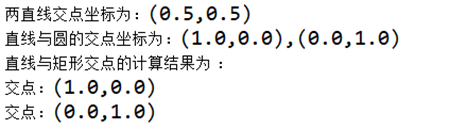第一次写博客，决定写博客是因为自己写程序时会出现很多不懂得地方，搞懂了后会很多的感想，于是就希望记录下来加深印象，同时希望这篇博客能给大家带来帮助！大家如果有什么更好的方法欢迎留言，谢谢！


展开全文java
• 直线和圆的交点公式 y=kx+b (x+c)² + (y+d)² = r² 的形式 转换成 ax+by+c=0 以（x, y）为圆心，r 为半径的形式 c++ // ax+by+c=0 // （x, y）为圆心，r 为半径 double x1_ = -(sqrt(pow(r, 2) * (1 + pow(a / b...
直线和圆的交点公式
y=kx+b
(x+c)² + (y+d)² = r²
的形式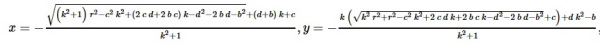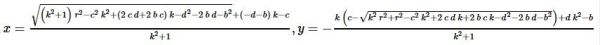转换成
ax+by+c=0
以（x, y）为圆心，r 为半径的形式
c++
// ax+by+c=0
// （x, y）为圆心，r 为半径
double x1_ = -(sqrt(pow(r, 2) * (1 + pow(a / b, 2)) - pow(x, 2) * pow(a / b, 2) -
(2 * x * y + 2 * c * x / b) * a / b - pow(y, 2) - 2 * c * y / b - pow(c / b, 2)) +
(y + c / b) * (a / b) - x) / (1 + pow(a / b, 2));
double y1_ = -(-a / b * (sqrt(pow(a / b, 2) * pow(r, 2) + pow(r, 2)
- pow(x, 2) * pow(a / b, 2) - x * x * y * a / b - x * x * a * c / pow(b, 2) -
pow(y, 2)
- 2 * c * y / b - pow(c / b, 2)) - x) - y * pow(a / b, 2) + c / b) /
(1 + pow(a / b, 2));
double x2_ = (sqrt(pow(r, 2) * (1 + pow(a / b, 2)) - pow(x, 2) * pow(a / b, 2) -
(2 * x * y + 2 * c * x / b) * a / b - pow(y, 2) - 2 * c * y / b - pow(c / b, 2)) -
(y + c / b) * (a / b) + x) / (1 + pow(a / b, 2));
double y2_ = -(-a / b * (-sqrt(pow(a / b, 2) * pow(r, 2) + pow(r, 2)
- pow(x, 2) * pow(a / b, 2) - x * x * y * a / b - x * x * a * c / pow(b, 2) -
pow(y, 2)
- 2 * c * y / b - pow(c / b, 2)) - x) - y * pow(a / b, 2) + c / b) /
(1 + pow(a / b, 2));


有需要根据第一个图片里的样子转换就可以了，或者用贴出的代码（有点乱）自己更改。
题目：直线和直线，直线和圆，直线和矩形的交点
GitHub：源码备份


展开全文• opencv2.4.9 & VS2013环境 ...2）霍夫直线检测可能会检测出多条重叠直线，利用上下两部分直线斜率相反筛选一下，选出两条直线。 3）两条直线求交点，变为解二元一次方程问题。 4）画出结果 实现： 1）
opencv2.4.9 & VS2013环境

问题描述

在图中找出两条直线，并找到两条直线交点位置。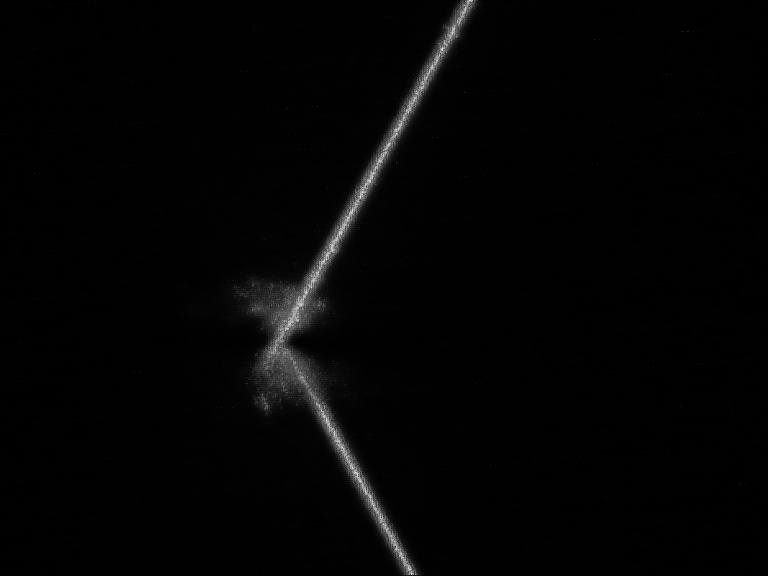思路：

1） 读图，二值化，简单腐蚀，之后直线在原图中比较明显，所以考虑直接用霍夫变换寻找直线。

2）霍夫直线检测可能会检测出多条重叠直线，利用上下两部分直线斜率相反筛选一下，选出两条直线。

3）两条直线求交点，变为解二元一次方程问题。

4）画出结果

实现：

1）调用opencv中的HoughLinesP函数：

void HoughLinesP( InputArray image, OutputArray lines,double rho, double theta, int threshold,double minLineLength=0, double maxLineGap=0 );

image为输入图像，要求是8位单通道图像

lines为输出的直线向量，每条线用4个元素表示，即直线的两个端点的4个坐标值

rho和theta分别为距离和角度的分辨率

threshold为阈值，即步骤3中的阈值

minLineLength为最小直线长度，在步骤5中要用到，即如果小于该值，则不被认为是一条直线

maxLineGap为最大直线间隙，在步骤4中要用到，即如果有两条线段是在一条直线上，但它们之间因为有间隙，所以被认为是两个线段，如果这个间隙大于该值，则被认为是两条线段，否则是一条。

2）检测出的直线为Vec4i类型，一共四位数。分别代表直线上的（x1,y1,x2,y2），求出每条直线写了，Ka * kB<0即代表两条直线鞋履相反

3）利用四个点坐标解方程，返回point2f类型的一个点

4）利用Line()以及circle()函数画出图像

代码：

/*Copyright@hitzym
Harbin Institute of Technology
Nov,29, 2017*/
# include <opencv2/opencv.hpp>
# include <iostream>

using namespace cv;
using namespace std;

/*函数功能：求两条直线交点*/
/*输入：两条Vec4i类型直线*/
/*返回：Point2f类型的点*/
Point2f getCrossPoint(Vec4i LineA, Vec4i LineB)
{
double ka, kb;
ka = (double)(LineA - LineA) / (double)(LineA - LineA); //求出LineA斜率
kb = (double)(LineB - LineB) / (double)(LineB - LineB); //求出LineB斜率

Point2f crossPoint;
crossPoint.x = (ka*LineA - LineA - kb*LineB + LineB) / (ka - kb);
crossPoint.y = (ka*kb*(LineA - LineB) + ka*LineB - kb*LineA) / (ka - kb);
return crossPoint;
}

int main(){

Mat src, grayImg, binImg, result;
//imshow("srcimage", src);
//waitKey(30);

/*检查图像是否载入*/
if (src.empty()) {
return -1;
}

/*转为灰度图*/
if (src.channels() == 3){
cvtColor(src, grayImg, CV_BGR2GRAY);
}
else if (src.channels() == 2){
grayImg = src.clone();
}

/*二值化*/
threshold(grayImg, binImg, 100, 255, THRESH_BINARY);
//imshow("binary image", binImg);
//waitKey(100);

/*腐蚀*/
Mat element = getStructuringElement(MORPH_RECT, Size(2, 1));
Mat erodeImg;
erode(binImg, erodeImg, element);
//imshow("erode", erodeImg);
//waitKey(100);

/*霍夫直线检测*/
vector<Vec4i> Lines;
HoughLinesP(erodeImg, Lines, 1, CV_PI / 360, 200, 100, 10);
Vec4i LineStand = Lines;
Vec4i LineAnother;
double ka = (double)(LineStand - LineStand) / (double)(LineStand - LineStand);
double kb;
for (int i = 1; i < Lines.size(); i++)
{
double ki = (double)(Lines[i] - Lines[i]) / (double)(Lines[i] - Lines[i]);
if (ki*ka < 0)
{
LineAnother = Lines[i];
kb = ki;
}
}

/*画出两条直线*/
result = src.clone();
line(result, Point(LineStand, LineStand), Point(LineStand, LineStand), Scalar(0, 255, 0), 2, 8);
line(result, Point(LineAnother, LineAnother), Point(LineAnother, LineAnother), Scalar(0, 0, 255), 2, 8);
cout << "直线A过点(" << LineStand << "," << LineStand << ")以及点(" << LineStand<<"，"<<LineStand << ")；斜率为：" << ka << endl;
cout << "直线B过点(" << LineAnother << "，" << LineAnother << ")以及点(" << LineAnother << "，" << LineAnother << ")；斜率为：" << kb << endl;

/*求交点并画点保存，result.jpg存储在工程目录下*/
Point2f crossPoint;
crossPoint = getCrossPoint(LineStand, LineAnother);
circle(result, crossPoint, 6, Scalar(255, 0, 0));
imwrite("./result.jpg", result);
cout << "交点坐标为：" << crossPoint << endl;
cout << "Copyrigth@zym" << endl;
imshow("result", result);
waitKey(100);
system("pause");

return 0;
}

运行结果：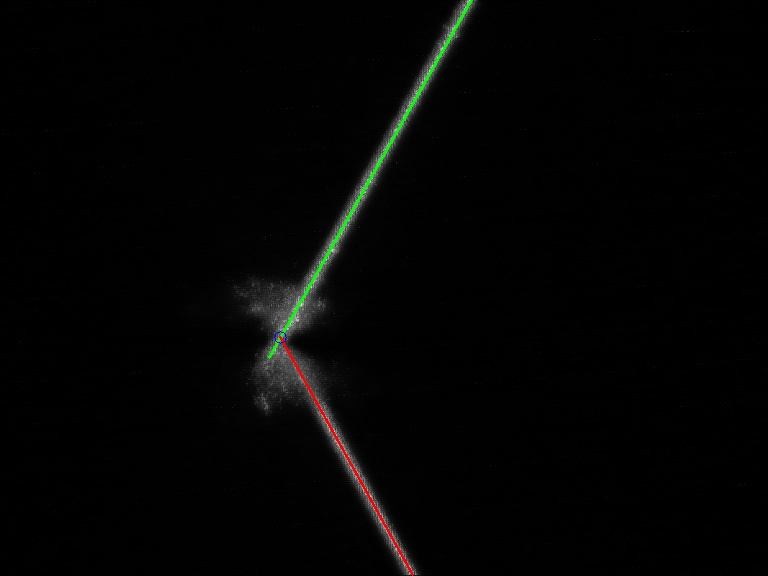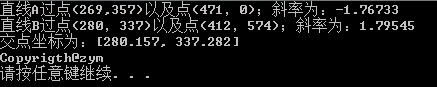一个很简单的小程序~
请各位看官多多指教！
展开全文opencv 图像处理
• python-opencv直线检测+直线拟合+计算偏移量 计算边界直线与x坐标轴的距离=首先canny边缘检测——>直线检测——>直线拟合——>计算与x坐标轴的距离。 直接上代码： import cv2 #opencv读取的格式是BGR ...python opencv
• ## 空间直线

千次阅读 2019-03-09 11:05:37
空间直线 空间直线由两个相交平面决定，方程组可表示这条直。 一般方程 点向式方程 又称对称式方程。 方向向量：平行于直线的向量。设（m,n,p），m,n,p为直线的一组方向数。 方向余弦：方向向量的余弦。 参数方程 ...高等数学 数学
• 点到线段的最短距离， 线段与线段的关系， 点到直线的距离， 直线直线的关系， 线段与直线的关系， 直线间的距离， 线段间的距离
• 斜率，亦称"角系数"，表示一条直线相对于横轴的倾斜程度。一条直线与某平面直角坐标系横轴正半轴方向的夹角的正切值即该直线相对于该坐标系的斜率。 如果直线与x轴垂直，直角的正切值无穷大，故此直线不存在斜率。 ...
• 已知直线上两点求直线的一般式方程 已知直线上的两点P1(X1,Y1) P2(X2,Y2)， P1 P2两点不重合。则直线的一般式方程AX+BY+C=0中，A B C分别等于： A = Y2 - Y1 B = X1 - X2 C = X2Y1 - X1Y2 直线外一点到直线的距离 P ...
• 题目：用面向对象的思维设计相关类，从而实现直线直线直线与圆、直线与矩形的交点。 菜鸡的C++实现： 公式： 直线直线：ax+bx+c=0; 一般公式：X = (c * otherline.b - b* otherline.c) /(b * otherline.a ...
• 直线检测 作业要求： 首先对测试图像（文件名为：test1~test6）进行边缘检测，可采用书上介绍的Sobel等模板或者cann算子方法； 在边缘检测的基础上，用hough变换检测图中直线； 比较不同边缘检测算法（2种以上）、...
• 利用点到直线距离 最小二乘法拟合直线 非矩阵算法算法 经验分享
• 直线度的检测一直是测量难题之一，尤其是在线检测直线度的方法，在线直线度测宽仪的测量精度能达到多少，本文小编就来介绍一下。 直线度属于形状公差中的一种。形状公差是指单一提取（实际）要素形状的允许变动量。...
• opencv3找直线并求任意两条直线交点，并显示出来，是直线交点，因为我把线段延长了。 求交点函数 /*函数功能：求两条直线交点*/ /*输入：两条Vec4i类型直线*/ /*返回：Point2f类型的点*/ Point2f getCrossPoint...
• C# 画（绘制）直线 C#如何画直线 C#绘制直线 WPF 画（绘制）直线 有源代码
• 在进行直线拟合算法中，一直使用最小二乘法，使用时间长了，也比较熟练了，但是在最近一次使用中，最小二乘法在拟合垂直或者接近垂直的直线时，效果不好；斜率很大，使用稳定性不好。查阅《Computer.Vision.A.Modern...
• 一、直线方程 点斜式：$$y-y_1=k(x-x_1)$$(其中$$l$$过定点$$P_1(x_1，y_1)$$，斜率为$$k$$)； 缺陷：不能表示斜率不存在的直线； 斜截式：$$y=kx+b$$($$k$$是斜率，$$b$$是$$y$$截距)； 缺陷：不能表示斜率...
• 背景介绍最近在水面无人艇(USV)模拟仿真中，用到了一些点和线的关系求解，本文主要讲述一下两点确认直线，点到直线距离，两条直线的交点等问题的解决方法，并给出python程序。部分内容非原创，文中给出链接，需要者...
• ## DDA直线算法

千次阅读 2019-09-21 21:29:03
简单来说就是画一条从(x1, y1)到(x2, y2)的直线，实质上是一个发现最佳逼近直线的像素序列，并填入色彩数据的过程。这过程也称为直线光栅化。 直线光栅化，首先我们要保证三个特点： 连续性 粗细、亮度要...
• ## 直线检测算法汇总

万次阅读 多人点赞 2019-09-21 20:15:07
目录1、场景需求2、Hough_line直线检测算法2.1 Hough_line实现步骤2.2 Hough_line代码实战2.3 效果展示与分析2.4 HoughP_line代码实战2.5 效果展示与分析3、LSD直线检测算法-[项目主页]...[论文链接]...
• 实验要求，生成5条直线并添加噪声，使用霍夫变换拟合直线 霍夫变换原理请参考此博客：https://blog.csdn.net/u010712012/article/details/86104053 实验代码 clc clear %生成五条标准直线用于直线检测% % x1=...MATLAB 霍夫变换
• python怎么画直线,画布,画图,创建一个,函数,对象python怎么画直线易采站长站，站长之家为您整理了python怎么画直线的相关内容。Tkinter是Python的标准 GUI 库。Python使用Tkinter可以快速的创建GUI应用程序。由于 ...
• 1、问题阐述 设xoyxoyxoy坐标系中一直线为： y=k∗x+b y=k*x+b y=k∗x+b 假设直线方向如图所示，计算直线在该方向上对应的向量。 2、求解
• 打开ps在界面新建一个...虽然这种方法是好，但是却只能横着或竖着画直线不能歪斜着画直线，那就用直线工具。如图。 右击路径的矩形工具，选择“直线工具”。如图。 然后在画布上斜着画一条直线，如图。 ...学习使我快乐
• Unity Curvy如何拉直线？ 什么插件可以拉直线？ 物体沿着直线unity